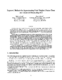## Improved Methods for Approximating Node Weighted Steiner Trees and Connected Dominating Sets

 dc.contributor.author Guha, Sudipto en_US dc.contributor.author Khuller, Samir en_US dc.date.accessioned 2004-05-31T22:48:36Z dc.date.available 2004-05-31T22:48:36Z dc.date.created 1997-12 en_US dc.date.issued 1998-10-15 en_US dc.description.abstract A greedy approximation algorithm based on spider decompositions'' was developed by Klein and Ravi for node weighted Steiner trees. This algorithm provides a worst case approximation ratio of $2 \ln k$, where $k$ is the number of terminals. However, the best known lower bound on the approximation ratio is $\ln k$, assuming that $NP \not\subseteq DTIME[n^{O(\log \log n)}]$, by a reduction from set cover. We show that for the unweighted case we can obtain an approximation factor of $\ln k$. For the weighted case we develop a new decomposition theorem, and generalize the notion of spiders'' to branch-spiders'', that are used to design a new algorithm with a worst case approximation factor of $1.5 \ln k$. This algorithm, although polynomial, is not very practical due to its high running time; since we need to repeatedly find many minimum weight matchings in each iteration. We are able to generalize the method to yield an approximation factor approaching $1.35 \ln k$. We also develop a simple greedy algorithm that is practical and has a worst case approximation factor of $1.6103 \ln k$. The techniques developed for the second algorithm imply a method of approximating node weighted network design problems defined by 0-1 proper functions. These new ideas also lead to improved approximation guarantees for the problem of finding a minimum node weighted connected dominating set. The previous best approximation guarantee for this problem was $3 \ln n$. By a direct application of the methods developed in this paper we are able to develop an algorithm with an approximation factor approaching $1.35 \ln n$. (Also cross-referenced as UMIACS-TR-97-80) en_US dc.format.extent 238697 bytes dc.format.mimetype application/postscript dc.identifier.uri http://hdl.handle.net/1903/924 dc.language.iso en_US dc.relation.isAvailableAt Digital Repository at the University of Maryland en_US dc.relation.isAvailableAt University of Maryland (College Park, Md.) en_US dc.relation.isAvailableAt Tech Reports in Computer Science and Engineering en_US dc.relation.isAvailableAt UMIACS Technical Reports en_US dc.relation.ispartofseries UM Computer Science Department; CS-TR-3849 en_US dc.relation.ispartofseries UMIACS; UMIACS-TR-97-80 en_US dc.title Improved Methods for Approximating Node Weighted Steiner Trees and Connected Dominating Sets en_US dc.type Technical Report en_US
##### Original bundle
Now showing 1 - 2 of 2Name:
CS-TR-3849.ps
Size:
233.1 KB
Format:
Postscript Files
Description: